# Controlling Neural Networks with Rule Representations

Deep neural networks (DNNs) provide more accurate results as the size and coverage of their training data increases. While investing in high-quality and large-scale labeled datasets is one path to model improvement, another is leveraging prior knowledge, concisely referred to as “rules” — reasoning heuristics, equations, associative logic, or constraints. Consider a common example from physics where a model is given the task of predicting the next state in a double pendulum system. While the model may learn to estimate the total energy of the system at a given point in time only from empirical data, it will frequently overestimate the energy unless also provided an equation that reflects the known physical constraints, e.g., energy conservation. The model fails to capture such well-established physical rules on its own. How could one effectively teach such rules so that DNNs absorb the relevant knowledge beyond simply learning from the data?

In “Controlling Neural Networks with Rule Representations”, published at NeurIPS 2021, we present Deep Neural Networks with Controllable Rule Representations (DeepCTRL), an approach used to provide rules for a model agnostic to data type and model architecture that can be applied to any kind of rule defined for inputs and outputs. The key advantage of DeepCTRL is that it does not require retraining to adapt the rule strength. At inference, the user can adjust rule strength based on the desired operation point of accuracy. We also propose a novel input perturbation method, which helps generalize DeepCTRL to non-differentiable constraints. In real-world domains where incorporating rules is critical — such as physics and healthcare — we demonstrate the effectiveness of DeepCTRL in teaching rules for deep learning. DeepCTRL ensures that models follow rules more closely while also providing accuracy gains at downstream tasks, thus improving reliability and user trust in the trained models. Additionally, DeepCTRL enables novel use cases, such as hypothesis testing of the rules on data samples and unsupervised adaptation based on shared rules between datasets.

The benefits of learning from rules are multifaceted:

• Rules can provide extra information for cases with minimal data, improving the test accuracy.
• A major bottleneck for widespread use of DNNs is the lack of understanding the rationale behind their reasoning and inconsistencies. By minimizing inconsistencies, rules can improve the reliability of and user trust in DNNs.
• DNNs are sensitive to slight input changes that are human-imperceptible. With rules, the impact of these changes can be minimized as the model search space is further constrained to reduce underspecification.

## Learning Jointly from Rules and Tasks

The conventional approach to implementing rules incorporates them by including them in the calculation of the loss. There are three limitations of this approach that we aim to address: (i) rule strength needs to be defined before learning (thus the trained model cannot operate flexibly based on how much the data satisfies the rule); (ii) rule strength is not adaptable to target data at inference if there is any mismatch with the training setup; and (iii) the rule-based objective needs to be differentiable with respect to learnable parameters (to enable learning from labeled data).

DeepCTRL modifies canonical training by creating rule representations, coupled with data representations, which is the key to enable the rule strength to be controlled at inference time. During training, these representations are stochastically concatenated with a control parameter, indicated by α, into a single representation. The strength of the rule on the output decision can be improved by increasing the value of α. By modifying α at inference, users can control the behavior of the model to adapt to unseen data.DeepCTRL pairs a data encoder and rule encoder, which produce two latent representations, which are coupled with corresponding objectives. The control parameter α is adjustable at inference to control the relative weight of each encoder.

## Integrating Rules via Input Perturbations

Training with rule-based objectives requires the objectives to be differentiable with respect to the learnable parameters of the model. There are many valuable rules that are non-differentiable with respect to input. For example, “higher blood pressure than 140 is likely to lead to cardiovascular disease” is a rule that is hard to be combined with conventional DNNs. We also introduce a novel input perturbation method to generalize DeepCTRL to non-differentiable constraints by introducing small perturbations (random noise) to input features and constructing a rule-based constraint based on whether the outcome is in the desired direction.

## Use Cases

We evaluate DeepCTRL on machine learning use cases from physics and healthcare, where utilization of rules is particularly important.

### Improved Reliability Given Known Principles in Physics

We quantify reliability of a model with the verification ratio, which is the fraction of output samples that satisfy the rules. Operating at a better verification ratio could be beneficial, especially if the rules are known to be always valid, as in natural sciences. By adjusting the control parameter α, a higher rule verification ratio, and thus more reliable predictions, can be achieved.

To demonstrate this, we consider the time-series data generated from double pendulum dynamics with friction from a given initial state. We define the task as predicting the next state of the double pendulum from the current state while imposing the rule of energy conservation. To quantify how much the rule is learned, we evaluate the verification ratio.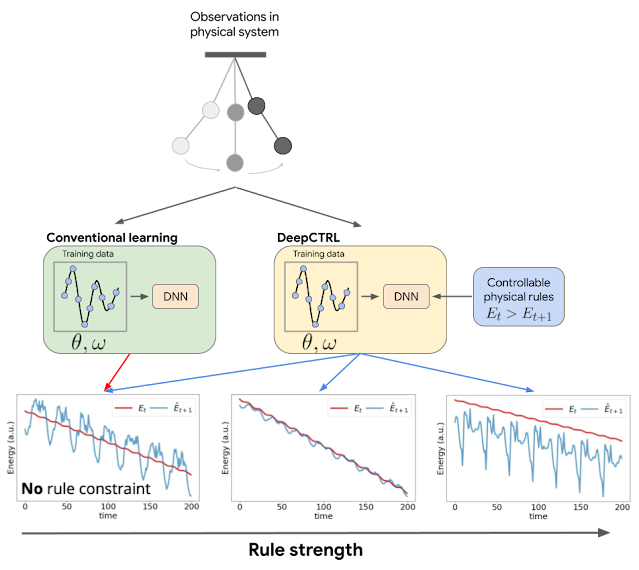DeepCTRL enables controlling a model's behavior after learning, but without retraining. For the example of a double pendulum, conventional learning imposes no constraints to ensure the model follows physical laws, e.g., conservation of energy. The situation is similar for the case of DeepCTRL where the rule strength is low. So, the total energy of the system predicted at time t+1 ( blue) can sometimes be greater than that measured at time t (red), which is physically disallowed (bottom left). If rule strength in DeepCTRL is high, the model may follow the given rule but lose accuracy (discrepancy between red and blue is larger; bottom right). If rule strength is between the two extremes, the model may achieve higher accuracy (blue curve is close to red) and follow the rule properly (blue curve is lower than red one).

We compare the performance of DeepCTRL on this task to conventional baselines of training with a fixed rule-based constraint as a regularization term added to the objective, λ. The highest of these regularization coefficients provides the highest verification ratio (shown by the green line in the second graph below), however, the prediction error is slightly worse than that of λ = 0.1 (orange line). We find that the lowest prediction error of the fixed baseline is comparable to that of DeepCTRL, but the highest verification ratio of the fixed baseline is still lower, which implies that DeepCTRL could provide accurate predictions while following the law of energy conservation. In addition, we consider the benchmark of imposing the rule-constraint with Lagrangian Dual Framework (LDF) and demonstrate two results where its hyperparameters are chosen by the lowest mean absolute error (LDF-MAE) and the highest rule verification ratio (LDF-Ratio) on the validation set. The performance of the LDF method is highly sensitive to what the main constraint is and its output is not reliable (black and pink dashed lines).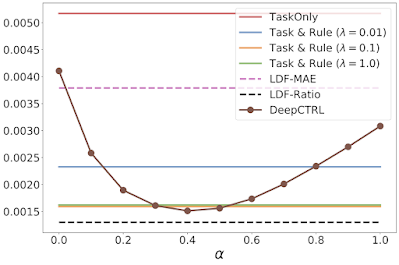Experimental results for the double pendulum task, showing the task-based mean absolute error (MAE), which measures the discrepancy between the ground truth and the model prediction, versus DeepCTRL as a function of the control parameter α. TaskOnly doesn’t have a rule constraint and Task & Rule has different rule strength (λ). LDF enforces rules by solving a constraint optimization problem.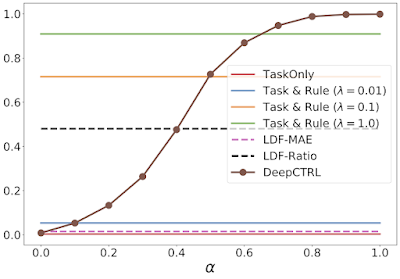As above, but showing the verification ratio from different models.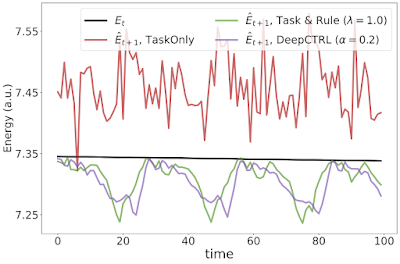Experimental results for the double pendulum task showing the current and predicted energy at time t and t + 1, respectively.

Additionally, the figures above illustrate the advantage DeepCTRL has over conventional approaches. For example, increasing the rule strength λ from 0.1 to 1.0 improves the verification ratio (from 0.7 to 0.9), but does not improve the mean absolute error. Arbitrarily increasing λ will continue to drive the verification ratio closer to 1, but will result in worse accuracy. Thus, finding the optimal value of λ will require many training runs through the baseline model, whereas DeepCTRL can find the optimal value for the control parameter α much more quickly.

### Adapting to Distribution Shifts in Healthcare

The strengths of some rules may differ between subsets of the data. For example, in disease prediction, the correlation between cardiovascular disease and higher blood pressure is stronger for older patients than younger patients. In such situations, when the task is shared but data distribution and the validity of the rule differ between datasets, DeepCTRL can adapt to the distribution shifts by controlling α.

Exploring this example, we focus on the task of predicting whether cardiovascular disease is present or not using a cardiovascular disease dataset. Given that higher systolic blood pressure is known to be strongly associated with cardiovascular disease, we consider the rule: “higher risk if the systolic blood pressure is higher”. Based on this, we split the patients into two groups: (1) unusual, where a patient has high blood pressure, but no disease or lower blood pressure, but has disease; and (2) usual, where a patient has high blood pressure and disease or low blood pressure, but no disease.

We demonstrate below that the source data do not always follow the rule, and thus the effect of incorporating the rule can depend on the source data. The test cross entropy, which indicates classification accuracy (lower cross entropy is better), vs. rule strength for source or target datasets with varying usual / unusual ratio are visualized below. The error monotonically increases as α → 1 because the enforcement of the imposed rule, which doesn’t accurately reflect the source data, becomes more strict.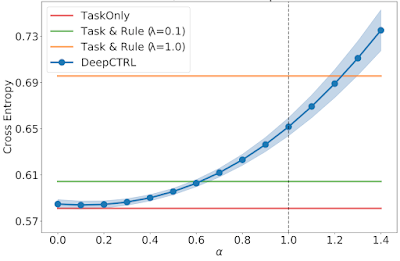Test cross entropy vs. rule strength for a source dataset with usual / unusual ratio of 0.30.

When a trained model is transferred to the target domain, the error can be reduced by controlling α. To demonstrate this, we show three domain-specific datasets, which we call Target 1, 2, and 3. In Target 1, where the majority of patients are from the usual group, as α is increased, the rule-based representation has more weight and the resultant error decreases monotonically.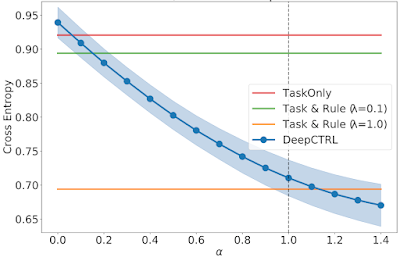As above, but for a Target dataset (1) with a usual / unusual ratio of 0.77.

When the ratio of usual patients is decreased in Target 2 and 3, the optimal α is an intermediate value between 0 and 1. These demonstrate the capability to adapt the trained model via α.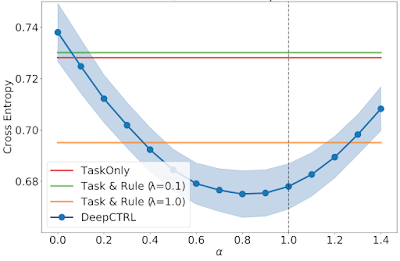As above, but for Target 2 with a usual / unusual ratio of 0.50.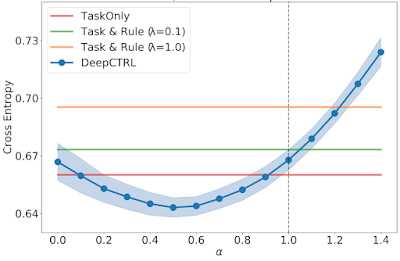As above, but for Target 3 with a usual / unusual ratio of 0.40.

## Conclusions

Learning from rules can be crucial for constructing interpretable, robust, and reliable DNNs. We propose DeepCTRL, a new methodology used to incorporate rules into data-learned DNNs. DeepCTRL enables controllability of rule strength at inference without retraining. We propose a novel perturbation-based rule encoding method to integrate arbitrary rules into meaningful representations. We demonstrate three use cases of DeepCTRL: improving reliability given known principles, examining candidate rules, and domain adaptation using the rule strength.

## Acknowledgements

We greatly appreciate the contributions of Jinsung Yoon, Xiang Zhang, Kihyuk Sohn and Tomas Pfister.